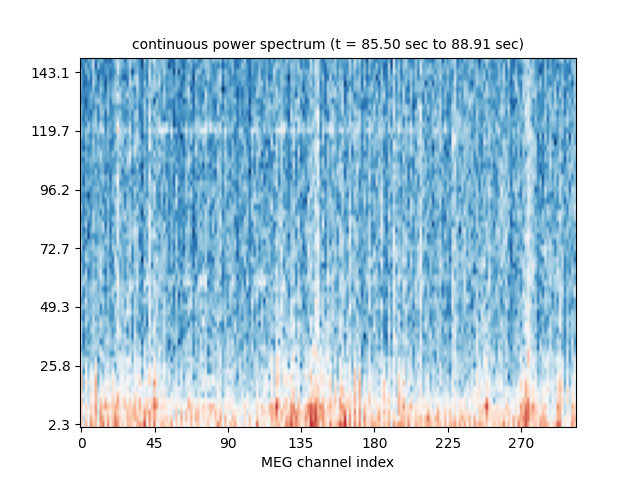# Compute real-time power spectrum density with FieldTrip client¶

Please refer to ftclient_rt_average.py for instructions on how to get the FieldTrip connector working in MNE-Python.

This example demonstrates how to use it for continuous computation of power spectra in real-time using the get_data_as_epoch function.```    Read a total of 3 projection items:
PCA-v1 (1 x 102)  idle
PCA-v2 (1 x 102)  idle
PCA-v3 (1 x 102)  idle
Running subprocess: neuromag2ft --file /home/circleci/mne_data/MNE-sample-data/MEG/sample/sample_audvis_raw.fif --speed 10
FieldTripClient: Waiting for server to start
Traceback (most recent call last):
File "/home/circleci/project/mne_realtime/externals/FieldTrip.py", line 227, in connect
self.sock = socket.socket(socket.AF_INET, socket.SOCK_STREAM)
FieldTripClient: Connected
1 matching events found
No baseline correction applied
0 projection items activated
Effective window size : 0.426 (s)
[Parallel(n_jobs=1)]: Using backend SequentialBackend with 1 concurrent workers.
[Parallel(n_jobs=1)]: Done   1 out of   1 | elapsed:    0.0s remaining:    0.0s
[Parallel(n_jobs=1)]: Done   1 out of   1 | elapsed:    0.0s finished
1 matching events found
No baseline correction applied
0 projection items activated
Effective window size : 0.426 (s)
[Parallel(n_jobs=1)]: Using backend SequentialBackend with 1 concurrent workers.
[Parallel(n_jobs=1)]: Done   1 out of   1 | elapsed:    0.0s remaining:    0.0s
[Parallel(n_jobs=1)]: Done   1 out of   1 | elapsed:    0.0s finished
1 matching events found
No baseline correction applied
0 projection items activated
Effective window size : 0.426 (s)
[Parallel(n_jobs=1)]: Using backend SequentialBackend with 1 concurrent workers.
[Parallel(n_jobs=1)]: Done   1 out of   1 | elapsed:    0.0s remaining:    0.0s
[Parallel(n_jobs=1)]: Done   1 out of   1 | elapsed:    0.0s finished
1 matching events found
No baseline correction applied
0 projection items activated
Effective window size : 0.426 (s)
[Parallel(n_jobs=1)]: Using backend SequentialBackend with 1 concurrent workers.
[Parallel(n_jobs=1)]: Done   1 out of   1 | elapsed:    0.0s remaining:    0.0s
[Parallel(n_jobs=1)]: Done   1 out of   1 | elapsed:    0.0s finished
1 matching events found
No baseline correction applied
0 projection items activated
Effective window size : 0.426 (s)
[Parallel(n_jobs=1)]: Using backend SequentialBackend with 1 concurrent workers.
[Parallel(n_jobs=1)]: Done   1 out of   1 | elapsed:    0.0s remaining:    0.0s
[Parallel(n_jobs=1)]: Done   1 out of   1 | elapsed:    0.0s finished
```

```# Author: Mainak Jas <mainak@neuro.hut.fi>
#

import os.path as op
import subprocess
import time

import numpy as np
import matplotlib.pyplot as plt

import mne
from mne.time_frequency import psd_welch
from mne.utils import running_subprocess

from mne_realtime import FieldTripClient

print(__doc__)

# user should provide info and list of bad channels because
# FieldTrip header object does not provide them
data_path = mne.datasets.sample.data_path()
'sample_audvis_raw.fif'))
bads = ['MEG 2443', 'EEG 053']

fig, ax = plt.subplots(1)

speedup = 10
command = ["neuromag2ft", "--file",
"{}/MEG/sample/sample_audvis_raw.fif".format(data_path),
"--speed", str(speedup)]
with running_subprocess(command, after='kill',
stdout=subprocess.PIPE, stderr=subprocess.PIPE):
with FieldTripClient(host='localhost', port=1972,
tmax=10, wait_max=5, info=info) as rt_client:

picks = mne.pick_types(info, meg='grad', eeg=False, eog=True,

n_fft = 256  # the FFT size. Ideally a power of 2
n_samples = 2048  # time window on which to compute FFT

# make sure at least one epoch is available
time.sleep(n_samples / info['sfreq'])

for ii in range(5):
epoch = rt_client.get_data_as_epoch(n_samples=n_samples,
picks=picks)
psd, freqs = psd_welch(epoch, fmin=2, fmax=200, n_fft=n_fft)

cmap = 'RdBu_r'
log_psd = 10 * np.log10(psd)

tmin = epoch.events / info['sfreq']
tmax = (epoch.events + n_samples) / info['sfreq']

if ii == 0:
origin='lower', cmap=cmap)

ax.set_yticks(np.arange(0, len(freqs), 10))
ax.set_yticklabels(freqs[::10].round(1))
ax.set_xlabel('Frequency (Hz)')
ax.set_xticks(np.arange(0, len(picks), 30))
ax.set_xticklabels(picks[::30])
ax.set_xlabel('MEG channel index')
im.set_clim()
else: